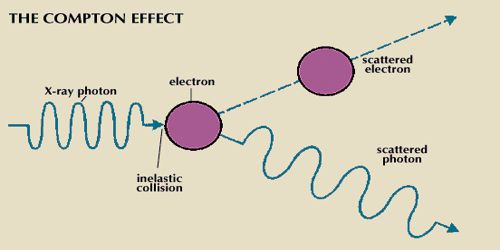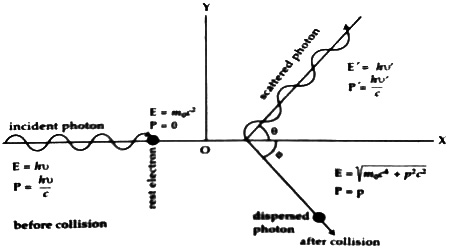Physics

# Compton EffectCompton Effect

After the establishment of the quantum theory of light, scientist Compton proposed in 1925 that if there is a collision between a powerful photon and a particle of matter electron, then the photon gives some energy to the electron. As a result, some amount of energy of the photon decreases. Due to the transfer of energy of photon the energy of the scattered photon becomes less than the energy of the incident photon. That means the wavelength of the scattered photon will be more than the wavelength of the incident photon. This change of wavelength is called Compton Effect. So, when a light wave (Photon) is occurrence on an electron, there is reduced in the energy of the photon as a part of energy its primary energy is transferred to the electron which is scattered. This effect is called the Compton Effect or Compton Scattering.Scientist Compton conserving the scattering process of the photon with electron and using the energies of photon and electron and applying conservation principle of momentum found out the change of frequency of wavelength of the photon. If monochromatic is scattered X-ray by the electron of light exerts e.g., carbon, aluminum etc. Then within the scattered rays there exists in addition to the incident wavelength X-rays of changed wavelengths are observed. These charged wavelengths are larger than the wavelength of the initial X-rays. In the figure, Compton Effect has been shown. The lost energy of photon due to collision is equal to the kinetic energy of an electron. The energy of the diffracted photon will be less than the energy of the incident photon. That means, the frequency of the scattered photon if will be less than the frequency of the incident photon ‘v’, (v – vˊ) 1). So, amount of energy gained by the electron is,

hv = hvˊ = Ek.

Here, h = Planck’s constant, Ek = kinetic energy of electron.

If the rest mass of the electron is m0 then according to the principle of conservation of energy, total energy before collision will be equal to the total energy after a collision. That means,

hv + m0c2 = hvˊ + √(m02c4 + p2c2)

[Incident photon energy + rest mass energy of the electron (before collision) = scattered photon energy + total energy of the scattered electron (after collision)]

In Compton Effect change of wave of incident photon (λ) and scattered photon (λˊ) is –

λˊ – λ = h/m0c (1 – cosθ)

In this equation by inserting the values of h, m0 and c Compton wavelength can be determined.

(h/m0c) is the wavelength of the scattered photon.

That means Compton wavelength, λc = (h/m0c) = [(6.63 x 10-34) J-s / (9.1 x 10-31 kg x 3 x 108 ms-1)] = 0.02468 x 10-10 m.